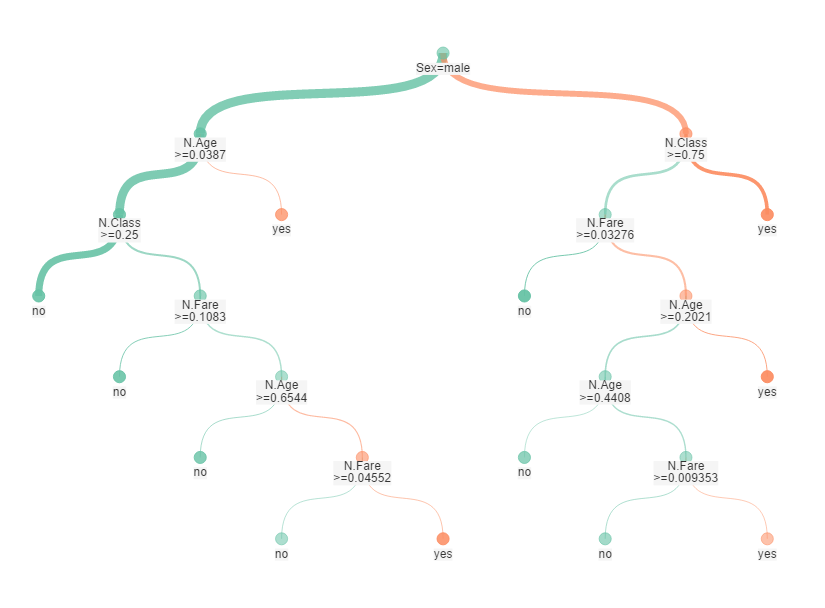## Alteryx Machine Learning Theory: Forest Model

by Jamie Gough

One of the major strengths of Alteryx is the ease with which it allows the user to run powerful predictive models. It can do this by merely attaching specified data to a predictive tool.

This blog will give a brief overview of a forest model, which is an extension of the classic decision tree model. Decision tree models transform each independent (predictor) variable into a classification question. A classification question has discrete answers, for example, what level of risk am I at for x disease (high, medium or low) or will this shot lead to a goal (yes or no).

Say if you wanted to predict the likelihood someone would have survived the Titanic or not – a decision tree can break down the variables age, sex, class and fare into classified questions. Once all these questions have been answered you can then calculate the chance of a person surviving. This is done by using the probability assigned to each answer. Below is how this decision tree would look in Alteryx.Although very logical, decision trees can lead to overfitting. Overfitting occurs when a model has too many independent variables that a very similar (correlated). This is a problem as the correlated variables will create a decision tree with similar questions. For example, if we wanted to predict if a university student will pass a test, using separate junior and senior school results would lead to a similar question being answered twice.

Forest models look to solve this problem by creating multiple trees with differing variables and classifications. There can be trees with only three variables as well as questions with different boundaries. An example of this, with the Titanic question, would be to have a tree that removes the age variable and changes the class question to a .5 – .5 split.

The logic behind this is pretty sound, by changing the variables and their classifications the model will be presented with a diverse group of predictions. The model will then combine these results to make the error less significant than if it were to use one tree.Jamie Gough

Thu 28 Sep 2017

Mon 04 Sep 2017

Fri 11 Aug 2017

Wed 09 Aug 2017